## Math 55a: Honors Abstract Algebra

Homework 3 <me@tylerlogic.com>

August 30, 2012
http://coursework.tylerlogic.com/math55a/homework03

Fine, I’ll give-in to the numbering system this time.

1 Implications of Spanning Set Countability

(a) Prove a vector space over a countable field that has a countable spanning set is countable as well.

Let V s = {v1,v2,} be the countable spanning set and F = {a1,a2,} be the countable field. Therefore for each vector vi in V s, the set of numbers ajvi is countable. In fact it is equivalent to the F itself. Thus the cardinality of the span of V s can not be more than the cardinality of the union of |V s| sets each with distinct elements and each of size |F|. Such a union would be countable , and therefore so is the vector span of which V s spans.

(b) Prove that any vector space with a countable spanning set over any field does not have an uncountable linearly independent set.

This is already proven to us via Axler’s Proposition 3.16 [1, pg 92] of chapter four.

2 Implications of Vector Space Countability on Spanning Sets

3 Problems 6 and 22 of Axler’s Third

(a) Problem 6

Let S1,S2Sn all be injective, linear mappings. Such that S1S2Sn is itself a mapping from, say V , to some arbitrary vector space. Therefore, if we have that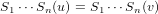for some vectors u,v V , then by injective property of S1 we also have the following.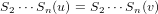Likewise by S2’s property of injection we also have that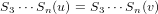and so on, until the continuation of this pattern arrives at the final implication that u = v. Hence S1Sn is an injection.

If S1Sn is injective, what can be said about each individual mapping? Each individual mapping is also injective in this case.

Assume that S1Sn is an injective mapping with some vector space V as its domain. Let S1Sn(u) = S1Sn(v) for some u,v V . From which we know that u = v by the injective property of S1Sn. Thus we have that Sn(u) = Sn(v) is also true, which in turn implies that that S1Sn-1 is injective. We can continue with this sequence of “if-this-than-that” n - 1 more times, arriving at the final conclusion that the mappings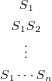are all injective.

Now assume that Si(u) = Si(v) for i ∈{1,,n} and some vectors u,vin the domain of Si. Therefore we have that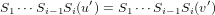which, by the above result, implies that u= v. Hence Si is an injective mapping, and subsequently, so are all the mappings S1,,Sn.

(b) Problem 22

Assume that V is a vector space and S,T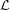(V ) are such that ST is invertible. Let T(u) = T(v) for some vectors u,v V . Therefore, ST(u) = ST(v) which implies that u = v since ST is an injection. Thus T is also an injection. Hence the by Axler’s Theorem 3.21 [2, pg 57], T is invertible.

In a similar manner, if we now assume that S(u) = S(v) then there exists a u,v′∈ V such that ST(u) = S(u) = S(v) = ST(v), by T’s surjectivity. Hence because ST is injective then u= v, which indicates that u = v since T is a bijection for which T(u) = u and T(v) = v are true. Thus S is an injection, for which Axler’s Theorem 3.21 [2, pg 57] yields to us that S is indeed invertible.

For the opposite direction, assume that S and T are each, individually, invertible mappings. From the solution to Axler’s problem six in chapter three (above) we learned that ST is therefore an injection. Thus, again using Axler’s Theorem 3.21 [2, pg 57], we have that ST too is invertible.

4 Problems 23 and 24 of Axler’s Third

(a) Problem 23

Let S,T(V ). Then assuming that we have ST = I then the following equation holds for some v V .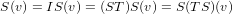Hence TS = I. The other direction is similarly proven by this proof due to symmetry.1

(b) Problem 24

Assume that T(V ) is a scalar multiple of the identity tranformation on(V ). Let this scalar be a. Hence for all S(V ) the following equation holds for some v V .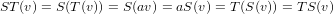Therefore we can see that ST = TS.

5 Polynomials on and Vector Spaces

6 Evaluation Map

(a) Show that the evaluation map is a linear transformation.

The following demonstrates the additivity of the evalutation map for some vectors L,S(V,W) and v V .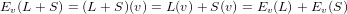With the following, we have homogeneity for some a in the field over which V and W lie.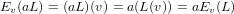Hence the evaluation map is a linear transformation.

7 Linear Maps and

Let V and W be vector spaces over the field of rationals, , and let T be a map from V to W. Proving additivty of T if T were to be linear is trivial; it’s one part of linearity, so we’ll simply assume additivity and prove homogeneity to gain linearity.

To aide in our proof let us first prove homogeneity when over Z. So assume that n . Then we have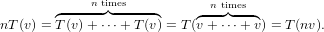(7.1)

Now let q =. Using equation 7.1 and a small trick, we get the following.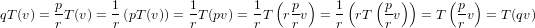Thus we have that T is linear.

8 Existence of Unique Dual Bases

I accidentally read through the part of the Wikipedia article, , that described the basis. Oh well, it’s kind of like conversing with a peer about the problem of which she already knows the answer, and she lets it slip.

Anyways, I realized after goin through the problem that if I just would have applied the “Kronecker-delta” result itself, the UNIQUE basis would have directly revealed itself.

Assume that V is a finite-dimensional vector space with v1,,vn as its basis, and that V * the vector space of linear maps from V to F, where F is the field over which V lies.

The existence and uniqueness of “Kronecker Maps” Let the mapping vj* in V *i for 1 through n be such that, for all v,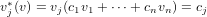then its easy to see that the jth of these mappings will each for the Kronecker map for each of the vectors in the basis of V mentioned above.

Assume by way of contradiction that for at least one j, vj* is not unique, taking ej V * to be one such mapping with ejvj* but ej(vi) = δij for the basis, v1,,vn. Therefore we have the following set of equations for each v V .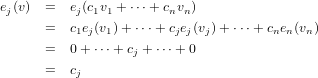Hence we have a contradiction and thus, there is no alternative to vj*; its unique!

These maps are a basis for the dual space V *. By the following sequence of equations for an arbitrary vector, e, in the dual space of V , we have that v1*,,vn* spans V *, if we let e(vi) = cifor each i of the indicies of the basis of V .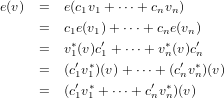Assume by way of contradiction that this set of vectors in the dual space is not linearly independent. Thus, at the very least, one of these vectors is a linear combination of the others. Let this said vector be vi*, which therefore implies that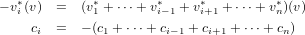for some vector v = c1v1 ++ cnvn V . However, this leads to the following set of equations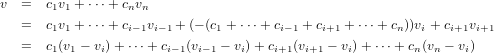in which we see that any vector v V is a linear combination of n- 1 vectors, which considering that V has dimension n is a contradiction. Hence we have that v1*,,vn* is a basis for the dual space, V *.

9 Dual of Direct Sum of Multiple Vector Spaces

What in the world is I?

10 Dual Bases in Fm+1

Let x0,x1,,xm each be distinct elements of F. Let A be m + 1 ×m + 1 matrix whose ith column is the ithvector of the set of vectors, vi := (x0i,x1i,,xmi). Since we have seen that this set of vectors forms a basis for Fm+1, then we know that the following holds for an arbitrary vector v, and some scalars cj.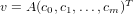This in turn yields to us the following.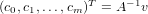Since the columns of A form a basis, by construction, then we know that it is invertible and that A-1 exists. Hence we can finally see that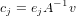where ej is simply the jth vector of the standard basis. Thus we are left to concluded that the jth element of the dual basis of Fm+1 is nothing more than the operation induced by multiplication by ejA-1.

### References

   Artin, Michael. Algebra. Prentice Hall. Upper Saddle River NJ: 1991.

   Axler, Sheldon. Linear Algebra Done Right 2nd Ed. Springer. New York NY: 1997.

   Rudin, Walter. Mathematical Analysis 3rd Ed. McGraw-Hill. New York NY: 1976.

   “Dual Space”, http://en.wikipedia.org/wiki/Dual_space.